Processing ......FreeComputerBooks.com Links to Free Computer, Mathematics, Technical Books all over the World

A Primer of Real Analysis
Top Free C++ Books 🌠 - 100% Free or Open Source!
• Title: A Primer of Real Analysis
• Author(s) Dan Sloughter
• Publisher: Furman University, August 26, 2018; eBook (Creative Commons Licensed)
• Paperback: N/A
• eBook: HTML and PDF (157 pages)
• Language: English
• ISBN-10/ASIN: B07GVQVMBB
• ISBN-13: N/ABook Description

This is a short introduction to the fundamentals of real analysis. Although the prerequisites are few, I have written the text assuming the reader has the level of mathematical maturity of one who has completed the standard sequence of calculus courses, has had some exposure to the ideas of mathematical proof (including induction), and has an acquaintance with such basic ideas as equivalence relations and the elementary algebraic properties of the integers.

• Dan Sloughter, Department of Mathematics, Furman University.
Reviews, Ratings, and Recommendations: Related Book Categories: Read and Download Links:Similar Books:
•Measure, Integration and Real Analysis (Sheldon Axler)

This textbook welcomes students into the fundamental theory of measure, integration, and real analysis. Focusing on an accessible approach, it lays the foundations for further study by promoting a deep understanding of key results.

•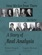How We Got from There to Here: A Story of Real Analysis

This book is an introductory real analysis textbook, presented through the lens of history. The definitions and techniques are motivated by the actual difficulties encountered by the intuitive approach and are presented in their historical context.

•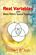Real Variables with Basic Metric Space Topology (Robert B. Ash)

Designed for a first course in real variables, this text presents the fundamentals for more advanced mathematical work, particularly in the areas of complex variables, measure theory, differential equations, functional analysis, and probability.

•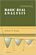Basic Real Analysis (Anthony W. Knapp)

This is a comprehensive treatment with a global view of the Real Analysis, emphasizing the connections between real analysis and other branches of mathematics. Included throughout are many examples and hundreds of problems.

•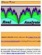Interactive Real Analysis (Bert G. Wachsmuth)

An interactive textbook for Real Analysis or Advanced Calculus in one real variable. It deals with sets, sequences, series, continuity, differentiability, integrability (Riemann and Lebesgue), topology, power series, and more.

•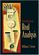Introduction to Real Analysis (William F. Trench)

Using an extremely clear and informal approach, this book introduces readers to a rigorous understanding of mathematical analysis and presents challenging math concepts as clearly as possible.

•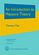An Introduction to Measure Theory (Terrence Tao)

This is a graduate text introducing the fundamentals of measure theory and integration theory, which is the foundation of modern real analysis, intended to cover a quarter or semester's worth of material for a first graduate course in real analysis.

Book Categories
 :All CategoriesTop Free BooksRecent BooksMiscellaneous BooksComputer EngineeringComputer LanguagesComputer ScienceData Science/DatabasesJava and Java EE (J2EE)Linux and UnixMathematicsMicrosoft and .NETMobile ComputingNetworking and CommunicationsSoftware EngineeringSpecial TopicsWeb Programming
Other Categories